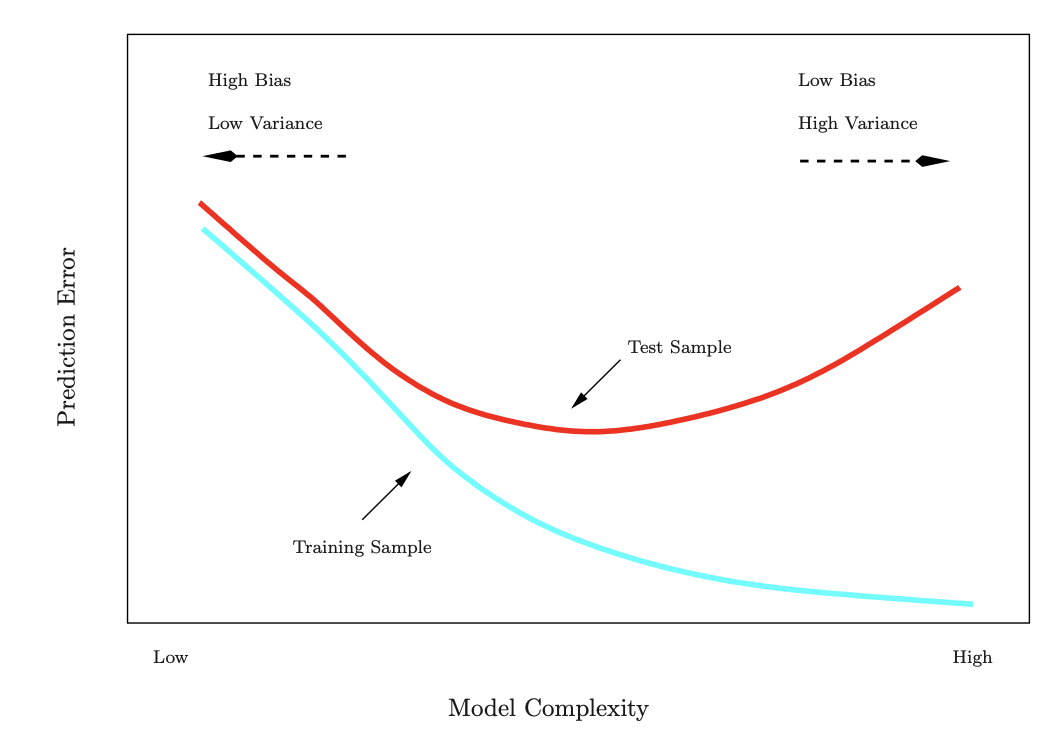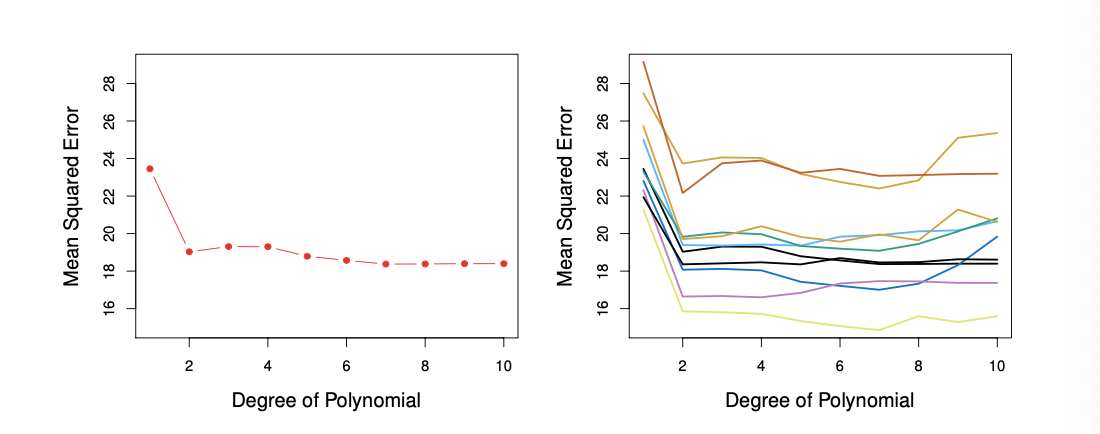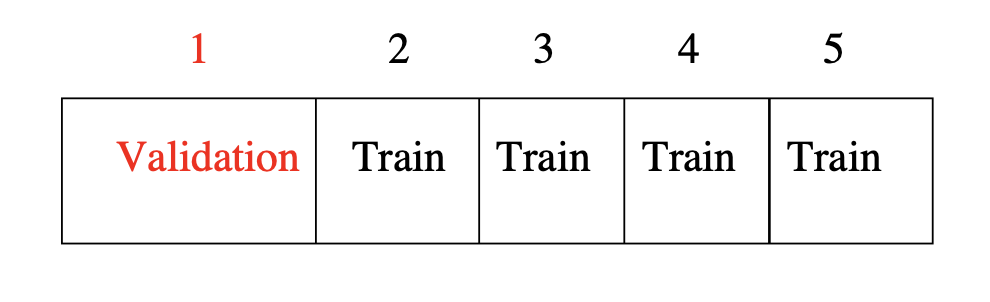## Resampling Methods

• Resampling methods are an indispensable tool in modern statistics

• They involve repeatedly drawing samples from a training set and refitting a model of interest on each sample in order to obtain additional information about the fitted model
• We will cover both cross-validation and the bootstrap

• Cross-validation can be used to estimate the test error associated with a given statistical learning method in order to evaluate its performance, or to select the appropriate level of flexibility

• The bootstrap is used in several contexts, most commonly to provide a measure of accuracy of a parameter estimate or of a given statistical learning method

• Model assessment

• The process of evaluating a model’s performance
• Model selection

• the process of selecting the proper level of flexibility for a model

## Cross-Validation and the Bootstrap

• We are going to discuss two resampling methods:

• Cross-validation

• Bootstrap

• These methods refit a model of interest to samples formed from the training set, in order to obtain additional information about the fitted model

• For example, they provide estimates of test-set prediction error, and the standard deviation and bias of our parameter estimates

## Training Error versus Test Error

• Recall the distinction between the test error and the training error:

• Test error

• Is the average error that results from using a statistical learning method to predict the response on a new observation, one that was not used in training the method
• Training error

• Can be easily calculated by applying the statistical learning method to the observations used in its training

• But the training error rate often is quite different from the test error rate, and in particular the former can dramatically underestimate the latter

• Training versus test performance## Validation-Set Approach

• Here we randomly divide the available set of samples into two parts: a training set and a validation or hold-out set

• The model is fit on the training set, and the fitted model is used to predict the responses for the observations in the validation set

• The resulting validation-set error provides an estimate of the test error. This is typically assessed using MSE in the case of a quantitative response and misclassification rate in the case of a qualitative (discrete) response

• Example

• Automobile data

• Want to compare linear vs higher-order polynomial terms in a linear regression

• We randomly split the 392 observations into two sets, a training set containing 196 of the data points, and a validation set containing the remaining 196 observations

• Left panel shows single split

• Right panel shows multiple splits• Drawbacks of validation set approach?

• the validation estimate of the test error can be highly variable, depending on precisely which observations are included in the training set and which observations are included in the validation set

• In the validation approach, only a subset of the observations — those that are included in the training set rather than in the validation set — are used to fit the model

• This suggests that the validation set error may tend to overestimate the test error for the model fit on the entire data set

• An example of cross-validation

library(tidyverse)
library(ISLR2)
library(rsample)

#set seed
set.seed(1234)

#data
Auto <- ISLR2::Auto

#Split the data into training and validation
split_auto  <- initial_split(Auto, prop = 0.5)
train_auto  <- training(split_auto)
test_auto   <- testing(split_auto)

#estimate a linear model
lm.fit <- lm(mpg ~ horsepower, data = train_auto)
summary(lm.fit)

#MSE
pred.fit <- predict(lm.fit, newdata = test_auto, type = "response")
mean((test_auto$mpg - pred.fit)^2) #Therefore, the estimated test MSE for the linear regression fit is 23.94 ### K-Fold Cross-Validation • Widely used approach for estimating test error • Estimates can be used to select best model, and to give an idea of the test error of the final chosen model • Idea is to randomly divide the data into $$K$$ equal-sized parts. We leave out part $$k$$, fit the model to the other $$K − 1$$ parts (combined), and then obtain predictions for the left-out $$kth$$ part • This is done in turn for each part $$k = 1, 2, ..., k$$ and then the results are combined • Example • Divide data into $$K$$ roughly equal-sized parts ($$K = 5$$ for this example)• Let the $$K$$ parts be $$C_1, C_2,...,C_k$$ denotes the indices of the observations in part $$k$$ • There are $$n_k$$ observations in part $$k$$: if $$N$$ is a multiple of $$K$$, then $$n_k = n/K$$ • Compute $$CV_{(K)} = \sum_{k=1}^{k} \frac{n_k}{n} MSE_k$$ • where $$MSE_k = \sum_{i \in C_k} (y_i - \hat{y_i})^2 / n_k$$ and $$\hat{y_i}$$ is the fit for observation $$i$$, obtained from the data with part $$k$$ removed • Setting $$K = n$$ yields $$n$$-fold or leave-one out cross validation (LOOVC) • With least-squares linear or polynomial regression, an amazing shortcut makes the cost of LOOCV the same as that of a single model fit! The following formula holds • $$CV_{(n)} = \frac{1}{n} \sum_{i=1}^{n} (\frac{y_i-\hat{y_i}}{1-h_i})^2$$ • Where $$y_i$$ is the $$ith$$ fitted value from the original least squares fit and $$h_i$$ is the leverage • This is like the ordinary MSE, except the $$ith$$ residual is divided by $$1 - h_i$$ • LOOCV is sometimes useful, but typically doesn’t shake up the data enough. The estimates from each fold are highly correlated and hence their average can have high variance • A better choice is $$K = 5$$ or 10 library(caret) ## Loading required package: lattice ## ## Attaching package: 'caret' ## The following object is masked from 'package:purrr': ## ## lift #set seed set.seed(1234) #data Auto <- ISLR2::Auto #specify the cross-validation method ctrl <- trainControl(method = "cv", number = 10) #fit a regression model and use k-fold CV to evaluate performance model.fit <- train(mpg ~ horsepower, data = Auto, method = "lm", trControl = ctrl) #view summary of k-fold CV print(model.fit) #view final model model.fit$finalModel

#view prediction for each fold
model.fit\$resample

## Other Issues with Cross-Validation

• Since each training set is only $$(K-1)/K$$ as big as the original training set, the estimates of prediction error will typically be biased upward

• This bias is minimized when $$K = n$$ (LOOCV), but this estimate has a high variance, as noted earlier

• $$K=5$$ or 10 provides a good compromise for this bias-variance trade-off

### Cross-Validation for Classification Problems

• We divide the data into $$K$$ roughly equal-sized parts $$C_1, C_2, ..., C_k$$

• Where $$C_k$$ denotes the indices of the observations in part $$k$$

• There are $$n_k$$ observations in part $$k$$: if $$n$$ is a multiple of $$k$$, then $$n_k = n/K$$

• Compute

• $$CV_{(K)} = \sum_{k=1}^{K} \frac{n_k}{n} Err_k$$

• Where $$Err_k = \sum_{i \in C_k} I(y_i \neq \hat{y_i}) / n_k$$
• The estimated standard deviation of $$CV_K$$ is

• $$\hat{SE}(CV_K = \sqrt{\frac{1}{K} \sum_{k=1}^{K} \frac{(Err_k-\bar{Err_k})^2}{K-1}}$$

• This is a useful estimate, but strictly speaking, not quite valid

## Cross-Validation: Right and Wrong

• Consider a simple classifier applied to some two-class data

• Starting with 5000 predictors and 50 samples, find the 100 predictors having the largest correlation with the class labels

• We then apply a classifier such as logistic regression, using only these 100 predictors

• How do we estimate the test set performance of this classifer?

• Can we apply cross-validation in step 2, forgetting about step 1?

• NO!

• This would ignore the fact that in Step 1, the procedure has already seen the labels of the training data, and made use of them. This is a form of training and must be included in the validation process

• It is easy to simulate realistic data with the class labels independent of the outcome, so that true test error =50%, but the CV error estimate that ignores Step 1 is zero

• Wrong Way